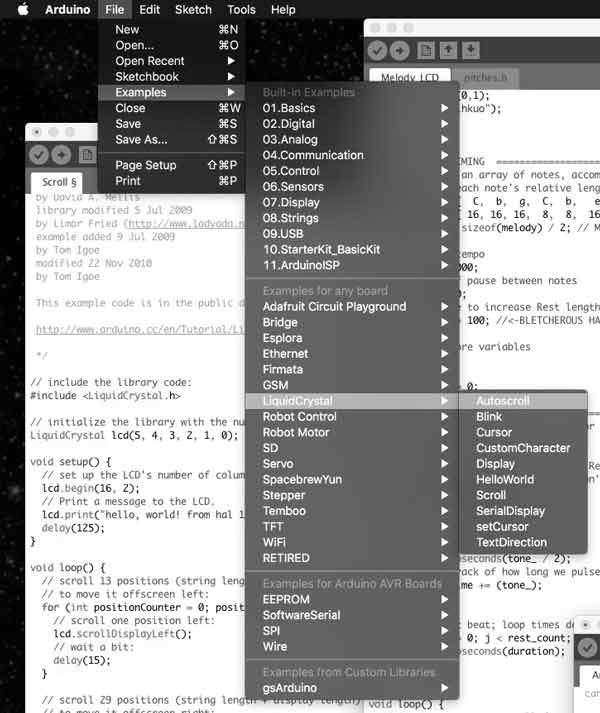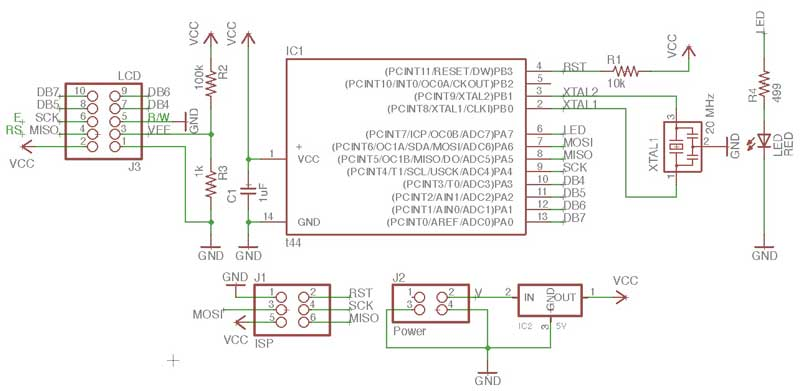# LCD x Arduino

Arduino has a built in libary, "LiquidCrystal.h", for programming this type of LCD. I thought it would be fun to imagine a prototype of HAL 9000 prior to artificial intelligence using the LCD display to communicate and the LED to hint life.

Arduino IDE is packaged with many sketches of LCD functions. Browse to File > Examples > LiquidCrystal and you can check those out.First, write in the sketch the command to send the library for the LCD.

`#include <LiquidCrystal.h>`

Then, you need to dictate which MCU pins are connected to the RS, E, DB4-7 pins of the LCD (in that order). That information can be found by taking a look back at the board schematic and the ATtiny -> Arduino pinout diagram.Write the code in the Arduino sketch as such, replacing the pin types with the pin numbers. The first box is the proper sequence, the second is the correct code for my board.

`LiquidCrystal lcd(RS, E, DB4, DB5, DB6, DB7);`
`LiquidCrystal lcd(5, 4, 3, 2, 1, 0);`

After examining and loading the examples to my board I made this modification of my breathing sketch and added some "dialogue" for HAL 900. I find that sending characters like this to the LCD, quickly exhausts the available programming memory. I think each character averages 1 byte of dynamic memory (maximum 256 bytes).

```#include <LiquidCrystal.h>

LiquidCrystal lcd(5, 4, 3, 2, 1, 0);

const int ledPin = 7;
int ledLevel = 255;
int timer = 30;
int ledChange = 15;
int ledMax = 255;
int ledMin = 0;
int ledPace = 5;

void setup() {
//LCD stuff
lcd.begin(16, 2);
lcd.setCursor(0,1);
lcd.print("HAL 900");
//LED stuff
pinMode(ledPin, OUTPUT);
ledMin = ledMax - (ledChange*(ledPace*2));
ledLevel = ledMax - (ledChange*ledPace);
}

void heartOne() {
while(ledLevel < ledMax) {
ledLevel = ledLevel + ledChange;
analogWrite(ledPin, ledLevel);
delay(timer);
}
while(ledLevel > (ledMin + (ledChange*ledPace))) {
ledLevel = ledLevel - ledChange;
analogWrite(ledPin, ledLevel);
delay(timer);
}
}

void heartTwo() {
while(ledLevel > ledMin) {
ledLevel = ledLevel - ledChange;
analogWrite(ledPin, ledLevel);
delay(timer);
}
while(ledLevel < (ledMax - (ledChange*ledPace))) {
ledLevel = ledLevel + ledChange;
analogWrite(ledPin, ledLevel);
delay(timer);
}
}

void loop() {
heartTwo();
heartOne();

lcd.clear();
lcd.setCursor(0,0);
lcd.print("/"I am putting");
lcd.setCursor(0,1);
lcd.print("myself to the...");

heartTwo();

lcd.clear();
lcd.setCursor(0,0);
lcd.print("fullest possible");
lcd.setCursor(0,1);
lcd.print("use, which is...");

heartOne();

lcd.clear();
lcd.setCursor(0,0);
lcd.print("all I think that");
lcd.setCursor(0,1);
lcd.print("any conscious...");

heartTwo();

lcd.clear();
lcd.setCursor(0,0);
lcd.print("entity can ever");
lcd.setCursor(0,1);
lcd.print("hope to do.\"");

heartOne();

lcd.clear();
lcd.setCursor(0,1);
lcd.print("HAL 900");
}```